# AP Physics C - Kinematics: Homeschool Curriculum Chapter Exam

Exam Instructions:

Choose your answers to the questions and click 'Next' to see the next set of questions. You can skip questions if you would like and come back to them later with the yellow "Go To First Skipped Question" button. When you have completed the practice exam, a green submit button will appear. Click it to see your results. Good luck!

### Page 1

#### Question 4 4. Using this graph, at what point is there a change in direction?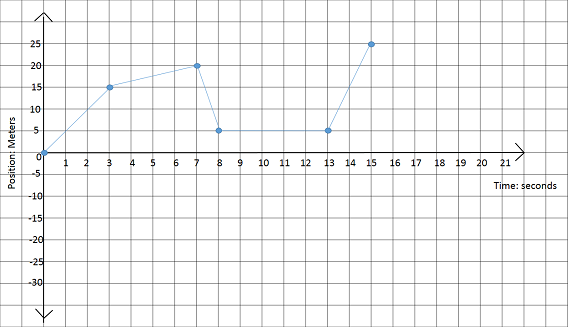#### Question 5 5. This graph is broken up into 5 sections, labeled A-E .On this graph, what section(s) represents negative acceleration?### Page 2

#### Question 6 6. For this graph, calculate the average velocity between 0 seconds and 3 seconds.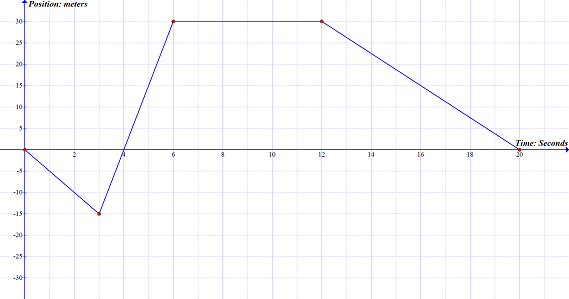#### Question 10 10. On this graph, what is the acceleration between 5 seconds and 8 seconds?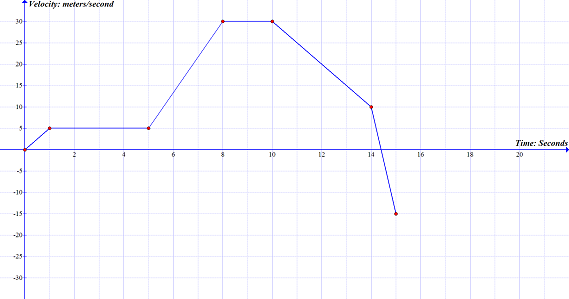### Page 3

#### Question 14 14. Using this graph, what is the distance of the object from the start point at 15 seconds?#### Question 15 15. On this graph, what is the acceleration between 10 seconds and 14 seconds?### Page 4

#### Question 18 18. For this graph, calculate the total average speed.#### Question 19 19. On this graph, what is the acceleration between 1 seconds and 5 seconds?### Page 5

#### Question 23 23. Calculate the slope of the line on the graph.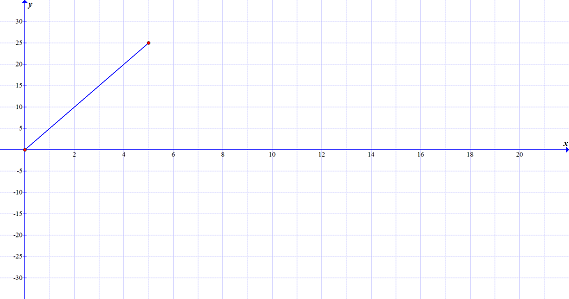#### Question 24 24. For this graph, calculate the average speed between 12 seconds and 20 seconds.### Page 6

#### Question 27 27. This graph represents an object that is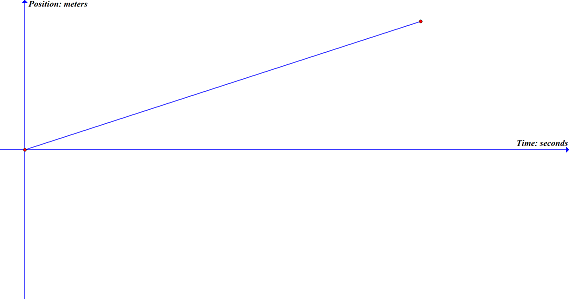#### Question 28 28. What key information is missing from the graph in this image?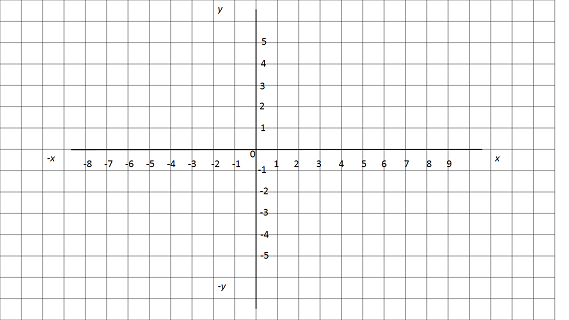#### Question 29 29. Calculate the displacement for some object in the diagram that starts at the red circle and ends at the blue triangle.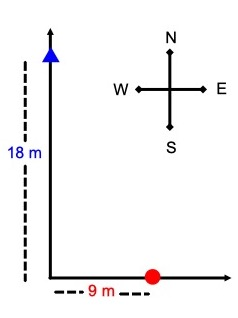#### AP Physics C - Kinematics: Homeschool Curriculum Chapter Exam Instructions

Choose your answers to the questions and click 'Next' to see the next set of questions. You can skip questions if you would like and come back to them later with the yellow "Go To First Skipped Question" button. When you have completed the practice exam, a green submit button will appear. Click it to see your results. Good luck!

Support